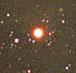The physical property that magnitude actually measures is radiant flux – the amount of light that arrives in a given area on Earth in a given time. Since color is measured by magnitude, a star’s color also depends on how much light arrives at Earth. Radiant flux is the physical basis for color.

The definition of magnitude m in terms of radiant flux F is:

`m = -log2.51(F/FVega).`

The star Vega in the northern hemisphere constellation Lyra is used as the standard for the magnitude system, so FVega means the amount of light arriving at Earth in a given time from Vega. This definition means that Vega’s magnitude is set at zero through all filters.

This does not mean that Vega looks the same through all filters; it just means that astronomers have agreed to use Vega as the zero point for the magnitude scale, much like the freezing point of water is used as the zero point for the Celsius temperature scale. There’s nothing special about Vega that made astronomers choose it as the zero point. They had to choose something… so why not Vega?

The negative sign in the definition ensures that brighter stars have smaller magnitudes. So if Earth receives less light from a certain star than from Vega (through a given filter), that star’s magnitude will be positive. If Earth receives more light from a certain star than from Vega, that star’s magnitude will be negative.Antares
Courtesy of the
Astronomical Image Data Archive.

Practice 1. The star Antares in the constellation Scorpius has a yellow magnitude of 1.2. How much of its light gets to Earth, as compared with Vega? The sun has a yellow magnitude of -26. How much of its light gets to Earth, as compared with Vega?

Remember that magnitude is a logarithmic quantity; a magnitude four star emits 2.51 times as much light as a magnitude five star. This allows you to define color in terms of the amount of light given off by a star.

A rule of logarithmic quantities, true for a logarithm with any base number (whether 10, e, or 2.51), is that log (x) – log (y) = log (x/y). Color is a difference in magnitudes; g-r is the difference between a star’s green magnitude and the same star’s red magnitude. Since magnitude is the log2.51 of radiant flux, you can express color in terms of radiant flux as g-r = -log2.51 (Fgreen) + log2.51 (Fred), which is equal to -log2.51 (Fgreen/Fred).

So if a star has a G-R color of 0.8, then

`-log2.51 (Fgreen/Fred) = 0.8,`

or

`log2.51 (Fgreen/Fred) = -0.8,`

which, by the definition of log, means that

`(Fgreen/Fred) = 2.51-0.8.`

Flip both sides of the equation to get:

`(Fred/Fgreen) = 2.510.8 = 2.08`

In other words, a star with G-R = 0.8 emits 2.08 times as much red-wavelength light as green-wavelength light.